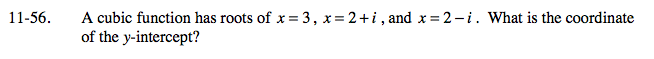Home > PC > Chapter 11 > Lesson 11.1.4 > Problem11-56

11-56.

A cubic function has roots of x = 3, x = 2 + i, and x = 2 − i. What is the coordinate of the y-intercept? Homework Help ✎Write the factors of the function.

y = (x − 3)(x − (2 + i))(x − (2 − i))

Let x = 0 to determine the y-intercept.